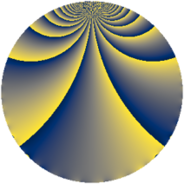# Properties

 Label 1210.2.kLevel $1210$ Weight $2$ Character orbit 1210.k Rep. character $\chi_{1210}(111,\cdot)$ Character field $\Q(\zeta_{11})$ Dimension $440$ Sturm bound $396$

# Related objects

## Defining parameters

 Level: $$N$$ $$=$$ $$1210 = 2 \cdot 5 \cdot 11^{2}$$ Weight: $$k$$ $$=$$ $$2$$ Character orbit: $$[\chi]$$ $$=$$ 1210.k (of order $$11$$ and degree $$10$$) Character conductor: $$\operatorname{cond}(\chi)$$ $$=$$ $$121$$ Character field: $$\Q(\zeta_{11})$$ Sturm bound: $$396$$

## Dimensions

The following table gives the dimensions of various subspaces of $$M_{2}(1210, [\chi])$$.

Total New Old
Modular forms 2020 440 1580
Cusp forms 1940 440 1500
Eisenstein series 80 0 80

## Trace form

 $$440q + 2q^{2} + 4q^{3} - 44q^{4} + 4q^{6} + 2q^{8} + 452q^{9} + O(q^{10})$$ $$440q + 2q^{2} + 4q^{3} - 44q^{4} + 4q^{6} + 2q^{8} + 452q^{9} + 2q^{10} + 12q^{11} - 18q^{12} - 32q^{13} - 32q^{14} - 36q^{15} - 44q^{16} + 28q^{17} + 26q^{18} + 16q^{19} + 48q^{21} + 10q^{22} + 32q^{23} + 4q^{24} - 44q^{25} + 20q^{26} + 64q^{27} + 40q^{29} + 4q^{30} - 56q^{31} + 2q^{32} + 44q^{33} + 20q^{34} + 8q^{35} - 32q^{36} + 16q^{37} + 12q^{38} + 48q^{39} - 20q^{40} + 40q^{41} + 16q^{42} + 32q^{43} + 12q^{44} + 12q^{46} + 32q^{47} + 4q^{48} - 104q^{49} + 2q^{50} + 14q^{51} + 12q^{52} - 100q^{53} + 16q^{54} + 12q^{55} + 12q^{56} + 58q^{57} - 72q^{58} + 56q^{59} + 8q^{60} + 56q^{61} + 24q^{62} + 96q^{63} - 44q^{64} + 20q^{65} + 26q^{66} - 24q^{67} + 28q^{68} + 112q^{69} + 64q^{71} + 26q^{72} - 12q^{73} + 36q^{74} + 4q^{75} - 72q^{76} - 8q^{77} + 32q^{78} - 52q^{79} + 520q^{81} - 44q^{82} + 96q^{83} + 48q^{84} - 104q^{85} + 12q^{86} + 152q^{87} - 56q^{88} + 8q^{89} - 62q^{90} - 176q^{91} - 100q^{92} - 36q^{93} - 44q^{94} + 4q^{96} - 56q^{97} - 94q^{98} + 156q^{99} + O(q^{100})$$

## Decomposition of $$S_{2}^{\mathrm{new}}(1210, [\chi])$$ into newform subspaces

The newforms in this space have not yet been added to the LMFDB.

## Decomposition of $$S_{2}^{\mathrm{old}}(1210, [\chi])$$ into lower level spaces

$$S_{2}^{\mathrm{old}}(1210, [\chi]) \cong$$ $$S_{2}^{\mathrm{new}}(121, [\chi])$$$$^{\oplus 4}$$$$\oplus$$$$S_{2}^{\mathrm{new}}(242, [\chi])$$$$^{\oplus 2}$$$$\oplus$$$$S_{2}^{\mathrm{new}}(605, [\chi])$$$$^{\oplus 2}$$